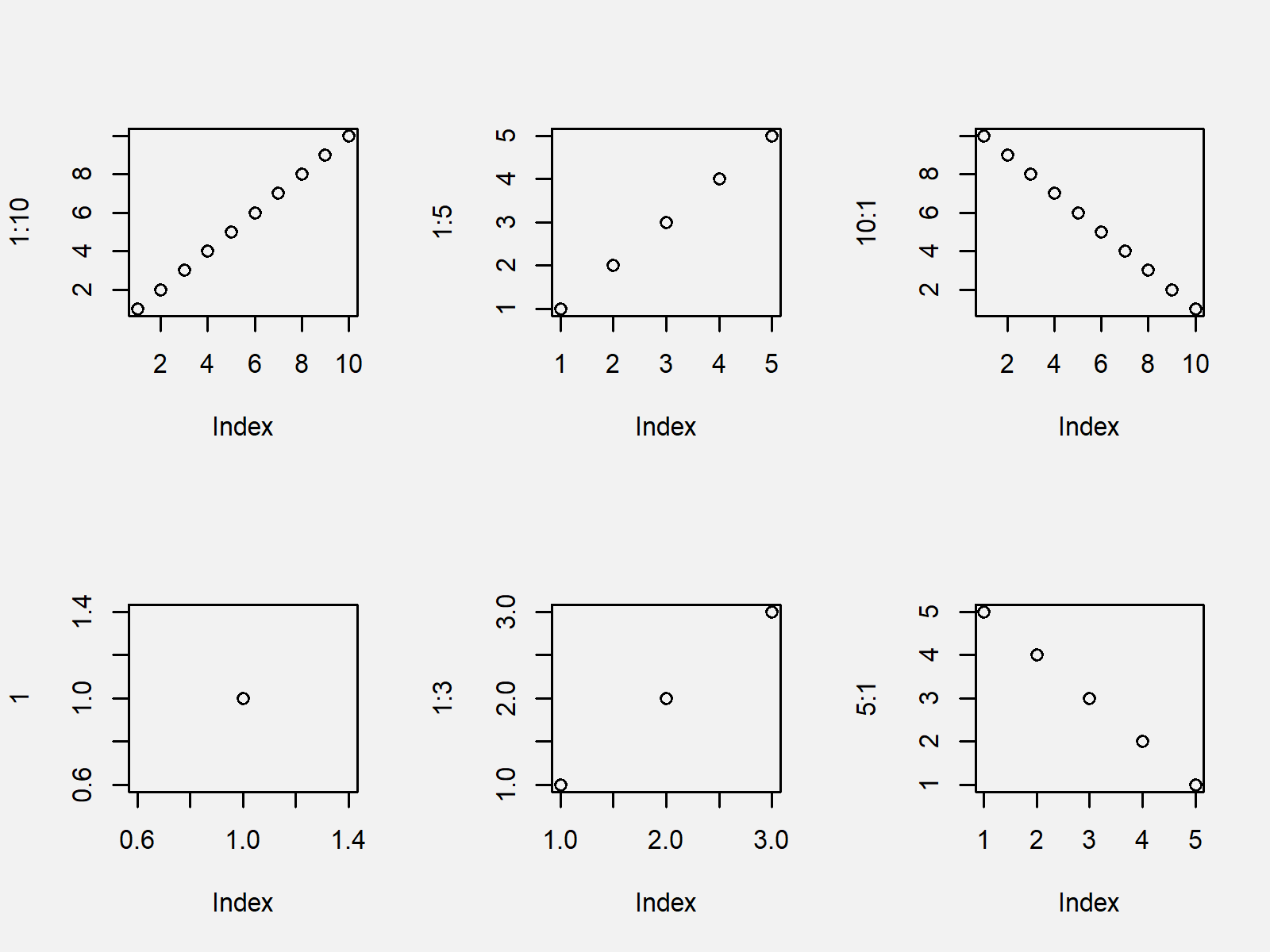# par Function in R (3 Examples)

In this R tutorial you’ll learn how to set or query graphical parameters using the par function.

Let’s dig in.

## Example 1: Create Graphic with Multiple Plots

In Example 1, I’ll illustrate how to draw a graphic containing multiple plot windows in R. For this task, we have to use the mfrow argument of the par function:

`par(mfrow = c(2, 3))              # Multiple plots`

Now, we can draw multiple plots in the same graphic:

```plot(1:10)                        # 1st plot
plot(1:5)                         # 2nd plot
plot(10:1)                        # 3rd plot
plot(1)                           # 4th plot
plot(1:3)                         # 5th plot
plot(5:1)                         # 6th plot```As you can see in Figure 1, the previous R code created a multi-plot graphic. The value 2 within the mfrow argument specified to draw a graphic with two rows and the value 3 specified to draw a graphic with three columns.

I recommend to set the par options back to their default values after you are finished with all your plots. Otherwise the changed par options will be kept until you restart RStudio. We can reset the par options using the dev.off() function:

`dev.off()                         # Set par options back to default`

## Example 2: Increase or Decrease White Space Around Borders of Plot

In this Example, I’ll explain how to change the size of the area around a plot by using the mar argument of the par function. We have to specify a vector of four values for the mar argument. The first value specifies the white space below the plot, the second value specifies the white space on the left side, the third value specifies the white space above the plot, and the fourth value specifies the white space on the right side.

`par(mar = c(5, 5, 10, 20))        # Change white space`

After running the par function with the previous specifications, we can draw any Base R plot we want:

`plot(1:10)                        # Draw plot`As shown in Figure 2, the previous syntax created a scatterplot with increased/decreased white space around the graph.

Again, let’s set the par options back to default so that we can continue with the next example:

`dev.off()                         # Set par options back to default`

## Example 3: Change Background Color of Plot

The code below illustrates how to change the background color of a plot using the par function and the bg argument. In this example, I’m setting the background color to yellow:

`par(bg = "yellow")                # Modify background`

Now, let’s draw another plot:

`plot(1:10)                        # Draw plot`As shown in Figure 3, the previous R syntax created a graphic with yellow background color.

Finally, let’s reset the par options:

`dev.off()                         # Set par options back to default`

## Video & Further Resources

Do you want to know more about the modification of graphical options? Then you might want to have a look at the following video of my YouTube channel. In the video, I explain the R code of this page.

Please accept YouTube cookies to play this video. By accepting you will be accessing content from YouTube, a service provided by an external third party.If you accept this notice, your choice will be saved and the page will refresh.

Furthermore, you might have a look at the related articles of my website. Some articles about graphics in R can be found below.